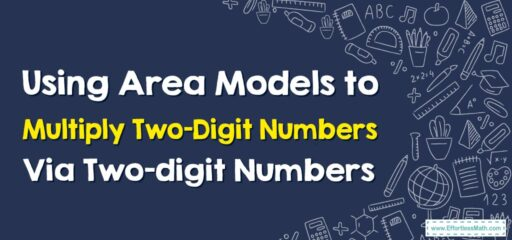# How to Use Area Models to Multiply Two-Digit Numbers By Two-digit Numbers

An area model is a visual tool that can help us understand the multiplication of two-digit numbers. It's based on the idea of breaking down each number into its tens and ones, then using a grid to represent and calculate the parts of the problem.## A Step-by-step Guide to Using Area Models to Multiply Two-Digit Numbers By Two-digit Numbers

Here’s a step-by-step guide to using area models to multiply two-digit numbers. For this example, we’ll use the problem $$23×45$$.

### Step 1: Break down the numbers into tens and ones

Break down each two-digit number into its tens and ones components. For example:

• 23 is $$20+3$$
• 45 is $$40+5$$

### Step 2: Draw the area model

Draw a rectangle and divide it into four sections. The width of the rectangle represents one of the numbers we are multiplying (23 in our example), and the height of the rectangle represents the other number (45). Divide the width into two sections (representing 20 and 3) and the height into two sections (representing 40 and 5).

It should look something like this:

  ---------------
|       |       |
|   20  |   3   |
|       |       |
|-------|-------|
|       |       |
|   40  |   5   |
|       |       |
---------------


### Step 3: Calculate the area of each section

Now, calculate the area of each of the four sections by multiplying the length and width of each section.

• The top left section represents 20 (from the width)$$\times40$$ (from the height) = 800.
• The top right section represents 3 (from the width) $$\times40$$ (from the height) = 120.
• The bottom left section represents 20 (from the width) $$\times5$$ (from the height) = 100.
• The bottom right section represents 3 (from the width) $$\times5$$ (from the height) = 15.

### Step 4: Add up all the areas

Finally, add up the four areas that you calculated:
$$800+120+100+15=1035$$
So, $$23×45=1035$$

The area model gives a visual representation of the multiplication problem, showing how each part of one number is multiplied by each part of the other number, and then how those products are added together to get the final result. It’s a great tool for understanding the concept of multiplication and the importance of place value.

### What people say about "How to Use Area Models to Multiply Two-Digit Numbers By Two-digit Numbers - Effortless Math: We Help Students Learn to LOVE Mathematics"?

No one replied yet.

X
30% OFF

Limited time only!

Save Over 30%

SAVE $5 It was$16.99 now it is \$11.99